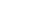• # Welcome!

Open or CloseWelcome to my DVD E-Store! This is the place to buy all my videos on disc!

New releases are at the

Click on a title below to get more information on each lesson. You can then add it to your order by clicking the "
Add to Cart" button. Click the "View Cart" button at bottom of page to finalize or make any changes to your order.

Note: The Basic Math lessons are for Pre-Algebra students or for those wanting to better prepare for the Algebra lessons.

Open or CloseThe DVDs are higher quality versions of the FREE videos on this web site. They contain NO advertising. You may download purchased videos to your iPod/iPhone/AppleTV or other video device that can play .M4V videos. If you are a teacher, you are allowed to use the purchased videos in your classroom, but may not place them onto your website.

The DVD-R discs should be compatible with most DVD players. The DVD-ROM folders/discs of the Math Lessons contain: videos in .M4V and possibly other formats, additional images, and PDF Math Notes.

Videos are in widescreen (16:9 aspect ratio) NOT standard (4:3 aspect ratio). DVDs are all in NTSC format (what TVs in North America and Japan use). They should all work well, if watched using a computer with DVD playing capability, so International Orders are welcome.

*Special Note to Teachers, Parents and Tutors: If you plan to use my videos for teaching/tutoring purposes, you would need to buy the DVDs (not just watch the free videos on this site with your students). The good news is no additional school licenses are needed!

Students: Although not required, it is appreciated if you could buy at least one DVD lesson from me.

Your purchases allow me to dedicate more time to creating even MORE and BETTER videos for you! Thank you in advance!

Privacy Policy: Any and all information you provide here will be kept private and will only be used to send you the items you purchased. I will not sell or use your private information in any other way.

Shipping Information: DVDs are sent via U.S. Postal Service. Please allow 2 days to 1 week for delivery in the U.S. and up to 2 weeks for International Orders. NOTE: Domestic orders are sent by First Class Mail or Priority Mail. International orders are sent either by First Class Air Mail or International Priority Mail. When I get your order, I will send you an acknowledgment email within a day (usually within a few minutes!) and another email message when the order is shipped.

Terms & Conditions: Defective DVD-R discs will be replaced at no charge within 10 days after you received item. I describe in detail what is covered in video, therefore, there are NO REFUNDS for DVDs purchased. You are responsible in deciding what you want.
• # Basic Math: Lesson 1 - Numbers

Open or Close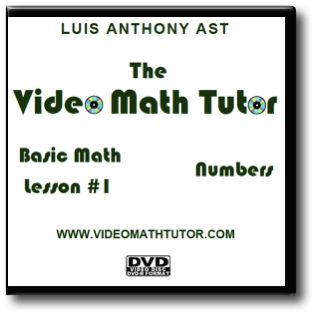Basic Math: Lesson 1 - Numbers

This lesson consists of providing you with a Self-Tutorial on all about the classification and sets of numbers. Learn what are natural numbers, integers, rational numbers and more. I also explain how to use your graphing calculator to input all types of numbers (integers, fractions, square roots, etc.).

39 minutes of video. 12 pages of printable notes. 1 DVD-R disc.

Price: \$12.99• # Basic Math: Lesson 2 - Equalities & Inequalities

Open or Close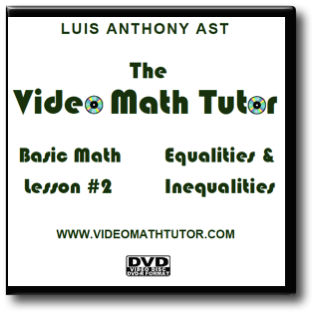Basic Math: Lesson 2 - Equalities & Inequalities

This lesson consists of providing you with a Self-Tutorial on the basics of equalities and inequalities. I go over how to write results in interval notation, inequality notation, and set (set-builder) notation. I also explain in the printed notes how to use your graphing calculator to help you make comparisons between numbers.

23 minutes of video. 10 pages of printable notes. 1 DVD-R disc.

Price: \$12.99• # Basic Math: Lesson 3 - Operations On Numbers

Open or Close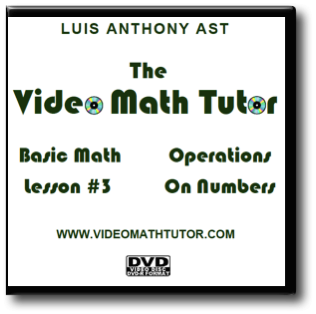Basic Math: Lesson 3 - Operations On Numbers

This lesson consists of providing you with a Self-Tutorial on the typical operations done on numbers. These include: absolute value, opposites, addition, subtraction, multiplication, division and simple exponents. Order of Operations is covered in detail. I also explain how to use your graphing calculator to help you perform all these operations.

125 minutes of video. 44 pages of printable notes. 2 DVD-R discs.

Price: \$19.99• # Basic Math: Lesson 4 - Properties of Numbers

Open or Close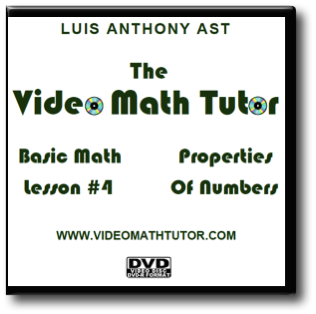Basic Math: Lesson 4 - Properties of Numbers

This lesson consists of providing you with a Self-Tutorial of the basic properties of numbers. These are the ones I discuss:

The Reflexive Property of Equality, The Symmetric Property of Equality, The Transitive Property of Equality, The Substitution Property, The Additive Property of Equality, The Cancellation Law of Addition, The Multiplicative Property of Equality, Cancellation Law of Multiplication, The Zero-Factor Property, The Law of Trichotomy, The Transitive Property of Inequality, Properties of Absolute Value, The Closure Property of Addition, The Closure Property of Multiplication, The Commutative Property of Addition, The Commutative Property of Multiplication, The Associative Property of Addition, The Associative Property of Multiplication, The Identity Property of Addition, The Identity Property of Multiplication, The Inverse Property of Addition, The Inverse Property of Multiplication, The Distributive Law of Multiplication Over Addition, The Distributive Law of Multiplication Over Subtraction, The General Distributive Property and The Negation Distributive Property.

38 minutes of video. 20 pages of printable notes. 1 DVD-R disc.

Price: \$12.99• # Basic Math: Lesson 5 - Factors, Multiples & Divisibility

Open or Close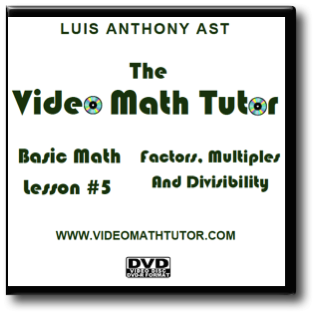Basic Math: Lesson 5 - Factors, Multiples & Divisibility

This lesson consists of giving you the necessary "preliminaries" to do fractions. The tutor explains what are factors, divisors, prime and composite numbers, how to do prime factorization, GCFs, LCMs, multiples, and the rules of divisibility. He also explains how to use your graphing calculator to help you find the GCF, LCM and multiples.

BONUS Lesson: Basic Math: Sieve of Eratosthenes

This lesson consists of showing you how to use the Sieve of Eratosthenes to find all prime numbers below a certain value. In this case, below 100.

52 minutes of video. 19 pages of printable notes. 1 DVD-R disc.

Price: \$15.99• # Basic Math: Lesson 6 - Fractions

Open or Close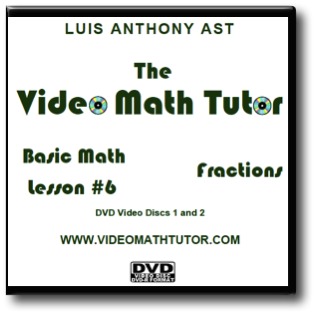Basic Math: Lesson 6 - Fractions

Lesson 6 consists of providing you with a Self-Tutorial on all about fractions. This lesson is different from the previous ones in that the use of printed Notes is integrated with the Video (other Notes may be used independently of the videos). This lesson is VERY detailed and covers the necessary areas to help students review the many aspects of fractions and fraction conversions. I also show you how to perform all the many different fraction operations and conversions using the TI-84+ Graphing Calculator. Here is how the lesson is broken down:

• An informal introduction to why we need to learn about fractions
• Understanding Fractions
• Types of Fractions
• Equivalent Fractions
• The LCD & Fraction Comparisons
• Other Fractional Expressions
• Converting Fractional Expressions
• Multiplication of Fractions
• Division of Fractions
• Subtraction of Fractions

220 minutes of video. 83 pages of printable notes. 4 DVD-R discs.

Price: \$24.99• # Basic Math: Lesson 7 - Units Of Measurement

Open or Close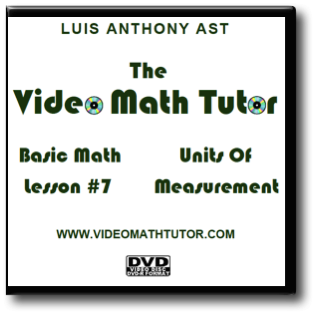Basic Math: Lesson 7 - Units Of Measurement

This lesson consists of providing you with a Self-Tutorial of the basic units used in measurement. These are the ones I discuss:
Units of Time, Units of Length (Metric and U.S.), Units of Area (Metric and U.S.), Units of Volume (Metric and U.S.), Units of Mass (Metric and U.S.), Units of Liquid Capacity (Metric and U.S.), Units of Dry Capacity (Metric and U.S.), and other miscellaneous Units. Conversion Formulas and Abbreviations are also listed.

This is a "must have" Lesson for those moving on to solving Word Problems in Algebra and other higher math.

32 minutes of video. 21 pages of printable notes. 1 DVD-R disc.

Price: \$12.99• # Algebra: Introduction To Algebra

Open or CloseAlgebra: Introduction To Algebra

This lesson consists of providing you with a Self-Tutorial on what is algebra, what are variables, constants, coefficients, terms, and expressions. The tutor explains the use of proper notation, how to combine like terms, find the negation of an algebraic expression, how to evaluate an expression (by hand and by using your calculator), and finally, there is a VERY detailed section on how to translate English phrases into algebraic expressions.

100 minutes of video. 43 pages of printable notes. 2 DVD-R discs.

Price: \$19.99• # Algebra: Formulas From Geometry

Open or CloseAlgebra: Formulas From Geometry

This lesson consists of providing you with a basic review of the formulas from geometry you will most likely encounter in algebra (and other math classes like trigonometry and calculus). This is NOT a detailed, formal lesson. Formulas and concepts covered include:

Angles (complementary & supplementary) • Triangles (perimeter and area) • Isosceles triangle • Equilateral triangle • Right triangle • The Pythagorean Theorem • Similar triangles • Perimeter and area formulas for Quadrilaterals (square, rectangle, parallelogram, trapezoid). Some diagonal formulas too, where applicable. • Circles (radius, diameter, circumference, area) • Cube (diagonal of face, diagonal of cube, surface area and volume) • Rectangular box [or Rectangular Parallelepiped] (diagonal of box, surface area and volume) • Prisms (Volume) • Pyramids (Volume) • Cone (slant height, lateral surface area, total surface area, volume) • Cylinder (lateral surface area, total surface area, volume) • Sphere (surface area and volume)

BONUS Lesson: Greek Alphabet. For use by math and science students, NOT for foreign language use.

23 minutes of video. 10 pages of printable notes. 1 DVD-R disc.

Price: \$12.99• # Algebra: Solving Linear Equations - Part 1: The Basics

Open or CloseAlgebra: Solving Linear Equations - Part 1: The Basics

Lesson consists of providing you with a Self-Tutorial on how to solve linear equations. Tutor discusses what is an Equation, Solution, Solution Set, Equivalent Equations, Identity, Contradictions, Conditional Equations, Linear Equations, the Standard Form of a Linear Equation. I show you how to actually SOLVE linear equations going step-by-step. I don't skip steps. I review some of the properties of Equality here too. I remind you to check the work done and how to solve linear equations using a calculator. At the end, you will know how to easily solve equations that look like:

2[y - (4y - 1)] = 5 - 9y

No word problems are done here. This is shown in DETAIL in Part 2 of the Lesson.

89 minutes of video. 34 pages of printable notes. 2 DVD-R discs.

Price: \$19.99• # Algebra: Solving Linear Equations - Part 2: Applications

Open or CloseAlgebra: Solving Linear Equations - Part 2: Applications

This lesson consists of providing you with a Self-Tutorial on how to solve typical linear word problems (story problems or applied problems). The tutor shows you how to solve for a specific variable in formulas. He also discusses how to covert a repeating decimal into a fraction (which was skipped in Basic Math: Lesson 6 -"Fractions") and will teach you how to convert units of measurement.

Examples of word problems done include:

Finding a number based on certain criteria.
Word problems involving some geometry (triangle, rectangle, circle).
Age problems.
Mixture problems.
Money problems (story of my life!).
Rate-Time-Distance problems.
Percent Equations/problems.
Ratio and Proportion (concepts and solving problems, including similar triangles).
Problems dealing with Unit Price.

180 minutes of video. 41 pages of printable notes. 4 DVD-R discs.

Price: \$29.99• # Video Brain Teasers Collection

Open or Close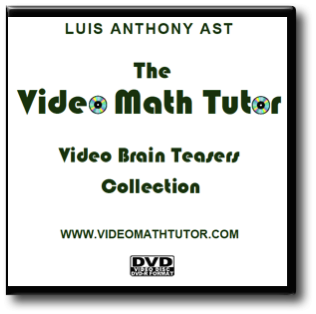Video Brain Teasers Collection

This is a collection of the first
58 Video Brain Teasers that have been posted here individually on this website (and elsewhere). These consist of recreational mental puzzles/problems dealing with the arrangement of matchsticks, coins, and other items. Also included is a pencil drawing game, a riddle, many Optical Illusions, a "Hidden Image" problem, a "Lateral Thinking" puzzle, and quite a few "Find the Twin" problems.

The puzzles on the Video DVD do not have the background music, only the individual puzzles in .M4V format have it in the DVD-ROM folder.

40 minutes of video. 18 pages of "Find the Twins" Puzzles in PDF Format. 1 DVD-R disc.

Price: \$14.99• # Algebra: Solving Linear Inequalities

Open or Close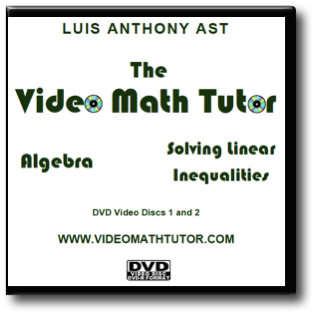Algebra: Solving Linear Inequalities

This lesson consists of providing you with a Self-Tutorial on how to solve typical linear inequalities of the form: ax + b > c (any of these symbols: <, >, ≤, ≥ can be used). The tutor goes over how to draw number line graphs. Also covered is the Double Inequality (a < x < b). Properties of Inequalities are reviewed. Answers are presented in Inequality Notation, Interval Notation, and Set Notation. Compound Linear Inequalities are solved and graphed. Finally, English Phrases are translated into inequalities and some Word Problems are solved.

142 minutes of video. 44 pages of printable notes. 3 DVD-R discs.

Price: \$24.99• # Video Flash Cards: Basic Fractions

Open or CloseVideo Flash Cards: Basic Fractions

This set of videos helps young students learn basic fractions using geometric shapes and other kid-friendly images. Pre-arranged sequences also provided for those too busy to make up their own. Fractions with numerators of zero are also included in this collection. Denominators covered: 1-10, 12, 14-16.

Two sizes of videos are used: one is 1280 x 720 pixels in MP4 format and is to be viewed full-screen on a computer monitor, iPad (or other tablet), Most Android devices or Apple TV, and one is 480 x 270 pixels in M4V format and is for use on an iPhone, iPod, or other similar portable video playing devices. 928 videos and image files of geometric shapes used to teach fractions are included, plus another 166 videos of other images are included on the DVD-ROM disc. Each Video Flash Card lasts 11.2 seconds.

3 hours, 20 minutes of video. 2 DVD-R discs.

Price: \$29.99• # Video Flash Cards: Five and Ten Frame

Open or CloseNEW! Video Flash Cards: Five and Ten Frame

Video Flash Cards to teach preschoolers and kindergarten students how to count using five and ten frames. I provide videos where frames are both horizontal and vertical. The dots in the frames appear in three versions: five-wise, pairwise, and subitized. There are Video Flash Cards that go from Frame to Number, and others that go from Number to Frame. There are over
270 videos in this collection. This is probably the greatest number of flash cards you will find anywhere!

BONUS MATERIAL:

• Six “Count the Dots” videos to teach kids to count using five and ten frames.
• A companion printout to use with videos (including a video explanation on how to use).
• 16 pre-made “random” sequences (of course, you are encouraged to make your own sequences with the individual flash cards).

All Video Flash Cards are 1920 by 1080 in MP4 format and can be viewed full-screen on a computer monitor or most mobile devices that can play video. Each Video Flash Card lasts approximately 15 seconds. Bonus videos last longer.

File Size: 1.1 GB

1 DVD-R disc.

Price: \$7.99